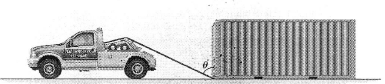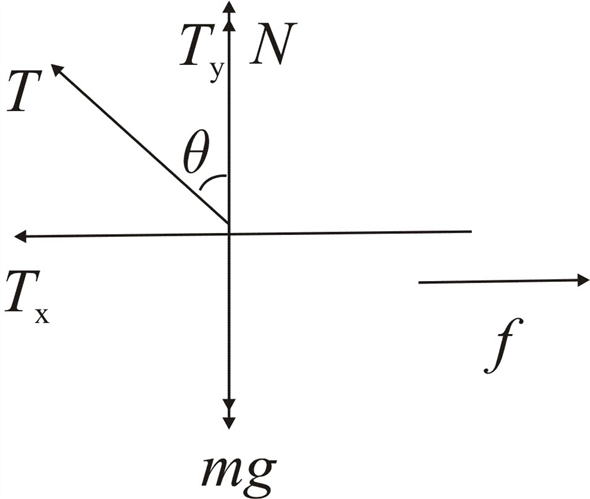Problem

# A tow truck of mass M is using a cable to pull a shipping container of mass m across a hor...

A tow truck of mass M is using a cable to pull a shipping container of mass m across a horizontal surface as shown in the figure. The cable is attached to the container at the front bottom corner and makes an angle θ with the vertical as shown. The coefficient of kinetic friction between the surface and the crate is μ.a) Draw a free-body diagram for the container.

b) Assuming that the truck pulls the container at a constant speed, write an equation for the magnitude T of the string tension in the cable.

#### Step-by-Step Solution

Solution 1

Think:

We can calculate the net force acting on the system by using Newton’s second law. In addition to the tension in the string, the forces on the container are weight of the container, normal force exerted by the ground on the container, and the frictional force acting in the opposite direction to the motion of the container.

Given data

Mass of the truck is $$M$$

Mass of the container is $$m$$

Angle made by the string with the vertical is $$\theta$$

Let the tension in the string be $$T$$, the frictional force be $$f$$ and the normal force be $$N$$.

(a) The tension of the string will be resolved into horizontal and vertical components. Then, the forces acting along x and y axis helps us to solve for tension in the string.

The free body diagram for the container is shown below,(b) Using Newton's second law, the net force acting on the container is calculated.

Net force on the container along $$x$$ axis is,

$$\begin{gathered} \sum F_{x}=m a_{x} \\ T_{x}-f=0 \\ T \sin \theta=\mu N \end{gathered}$$

Then normal force is

$$N=\frac{T \sin \theta}{\mu} \ldots \ldots(1)$$

Net force on the container along $$y$$ axis is,

$$\begin{gathered} \sum F_{y}=m a_{y} \\ N+T_{y}-m g=0 \\ T \cos \theta=m g-N \ldots \ldots \text { (2) } \end{gathered}$$

Substitute the value of normal force from equation (1) in equation (2), we get

\begin{aligned} T \cos \theta &=m g-\left(\frac{T \sin \theta}{\mu}\right) \\ \mu T \cos \theta+T \sin \theta &=\mu m g \\ T(\mu \cos \theta+\sin \theta) &=\mu m g \end{aligned}

Therefore the tension in the string is

$$T=\frac{\mu m g}{\mu \cos \theta+\sin \theta}$$